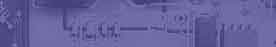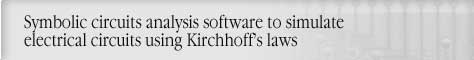Frequency, Period, Phase Angle of sinusoidal WaveformCircuit analysis Tutorial
AKNM Circuit Magic- circuit analysis software

Frequency, Period, Phase Angle of sinusoidal Waveform

The period of a waveform is the time required for completing one full cycle. It is measured in secondsFrequency of a Waveform

The frequency of a waveform is the number of cycles that is completed each second. It is measured in Hertz(Hz)

Phase Angle

The phase angle of a waveform is angular difference between two waveforms of the same frequency. It is measured in degrees or radians